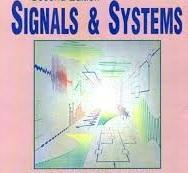# Signal And Systems Set 1

Approved & Edited by ProProfs Editorial Team
At ProProfs Quizzes, our dedicated in-house team of experts takes pride in their work. With a sharp eye for detail, they meticulously review each quiz. This ensures that every quiz, taken by over 100 million users, meets our standards of accuracy, clarity, and engagement.
| Written by Rejimano
R
Rejimano
Community Contributor
Quizzes Created: 2 | Total Attempts: 235
Questions: 10 | Attempts: 142SettingsSignal and Systems Set 1
This Quiz contains 10 Questions

• 1.

### The system described by the equation y(n)=ay(n-1)+b x(n) is a recursive system.

• A.

True

• B.

False

A. True
Explanation
Explanation: Since the present output depends on the value of the previous output, the system is called as Recursive system.

Rate this question:

• 2.

### Which of the following electromagnetic signals has a frequency range of 30kHz-3MHz?

• A.

• B.

• C.

• D.

Infrared signal

Explanation
Explanation: Radio broadcast signal is an electromagnetic signal which has a frequency range of 30kHz-3MHz.

Rate this question:

• 3.

### What is the frequency range(in Hz) of Electroencephalogram(EEG)?

• A.

10-40

• B.

1000-2000

• C.

0-100

• D.

None of the mentioned

C. 0-100
Explanation
Explanation: Electroencephalogram(EEG) signal has a frequency range of 0-100 Hz.

Rate this question:

• 4.

### The output signal when a signal x(n)=(0,1,2,3) is processed through an ‘Identical’ system is:

• A.

(3,2,1,0)

• B.

(1,2,3,0)

• C.

(0,1,2,3)

• D.

None of the mentioned

C. (0,1,2,3)
Explanation
An identical system is a system whose output is same as the input, that is it does not perform any operation on the input and transmits it.

Rate this question:

• 5.

### If a signal x(n) is passed through a system to get an output signal of y(n)=x(n+1), then the signal is said to be:

• A.

Delayed

• B.

• C.

No operation

• D.

None of the mentioned

D. None of the mentioned
Explanation
Explanation: For example, the value of the output at the time n=0 is y(0)=x(1), that is the system is advanced by one unit.

Rate this question:

• 6.

### The term ‘bandwidth’ represents the quantitative measure of a signal.

• A.

True

• B.

False

A. True
Explanation
Explanation: In addition to the relatively broad frequency domain classification of signals, it is often desirable to express quantitatively the range of frequencies over which the power or energy density spectrum is concentrated. This quantitative measure is called the ‘bandwidth’ of a signal.

Rate this question:

• 7.

### In general, a digital system designer has better control of tolerances in a digital signal processing system than an analog system designer who is designing an equivalent analog system.

• A.

True

• B.

False

A. True
Explanation
Analog signal processing operations cannot be done very precisely either, since electronic components in analog systems have tolerances and they introduce noise during their operation. In general, a digital system designer has better control of tolerances in a digital signal processing system than an analog system designer who is designing an equivalent analog system.

Rate this question:

• 8.

### If x(n) is a stable sequence so that X(z) converges on to a unit circle, then the complex cepstrum signal is defined as:

• A.

X(ln X(z))

• B.

Ln X(z)

• C.

X-1(ln X(z))

• D.

None of the mentioned

C. X-1(ln X(z))
Explanation
Let us consider a sequence x(n) having a z-transform X(z). We assume that x(n) is a stable sequence so that X(z) converges on to the unit circle. The complex cepstrum of the signal x(n) is defined as the sequence cx(n), which is the inverse z-transform of Cx(z), where Cx(z)=ln X(z)
=> cx(z)= X-1(ln X(z))

Rate this question:

• 9.

### Which of the following condition is to be satisfied for the Fourier transform of a sequence to be equal as the Z-transform of the same sequence?

• A.

|z|=1

• B.

|z|

• C.

|z|>1

• D.

Can never be equal

A. |z|=1
Explanation
Let us consider the signal to be x(n)

Rate this question:

• 10.

### What is the impulse response of the system described by the second order difference equation y(n)-3y(n-1)-4y(n-2)=x(n)+2x(n-1)?

• A.

[-1/5 (-1)n-6/5 (4)n]u(n)

• B.

[1/5 (-1)n – 6/5 (4)n]u(n)

• C.

[ 1/5 (-1)n+ 6/5 (4)n]u(n)

• D.

[- 1/5 (-1)n+ 6/5 (4)n]u(n)

D. [- 1/5 (-1)n+ 6/5 (4)n]u(n)
Explanation
The homogenous solution of the given equation is yh(n)=C1(-1)n+C2(4)n—-(1)
To find the impulse response, x(n)=δ(n)
now, for n=0 and n=1 we get
y(0)=1 and
y(1)=3+2=5
From equation (1) we get
y(0)=C1+C2 and
y(1)=-C1+4C2
On solving the above two set of equations we get
C1=- 1/5 and C2= 6/5
=>h(n)= [-1/5 (-1)n + 6/5 (4)n]u(n).

Rate this question:

Related TopicsBack to top
×

Wait!
Here's an interesting quiz for you.